## 3.3Finite volume mesh

The ﬁnite volume numerical method is closely associated with the concept of surface and volume integrals used in Chapter 2 . Below, we extract the main geometric elements from the ﬁgures used for the conservation laws.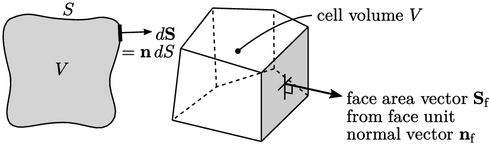The integrals use volumes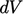and area vectors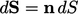. The numerical method uses equivalent discrete quantities for cells and faces:

• cell volume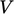;
• face area vector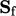, with area magnitude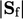;
• face unit normal vector.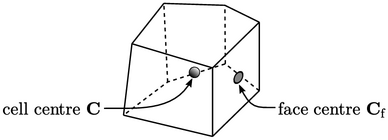The ﬁnite volume method relates discrete values of ﬁelds, e.g. pressure, to cells and faces within the mesh. For many calculations data must to be assigned to point locations, in particular the cell centre (more speciﬁcally centroid)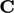and face centre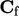.

### Calculating mesh data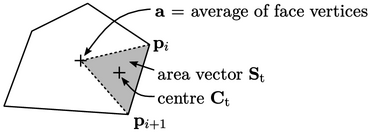To calculate, each polygonal face is decomposed into triangles using an apex point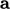. The area vector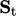and centre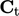are then calculated for each triangle according to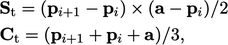where “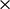” is the cross product, Eq. (2.70 ). The sum of area vectors gives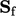and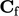is the area weighted sum of triangle centres over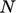triangles, calculated by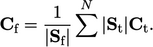The cell volume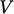can be calculated fromandby Gauss’s theorem, using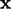to describe position and noting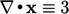. The surface integral (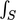) becomes a sum over cell faces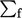, replacing discrete values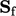and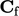for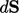and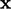respectively as follows: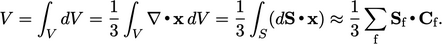The cell centre is calculated similarly, noting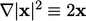, by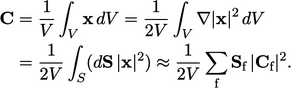Notes on CFD: General Principles - 3.3 Finite volume mesh